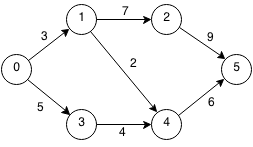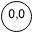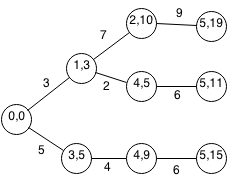## Introduction

Prerequisites: Shortest Path

Bellman Ford is an algorithm that finds the shortest path from one source node to every other node in the graph. The running time is O(n2) and is able to find negative cycles.

## Implementation

Bellman Ford can be done using backtracking to find the shortest path in a graph. We first start at the starting node with a starting cost of 0 and 0 edges used. For each node thats connected to that node, we repeat and add to the cost of the node.We will do an example of the Bellman Ford algorithm on the above graph. At each node we have the node index and the current weight to reach that node. We start at node 0 with a weight of 0.From node 0, we can reach node 1 and node 3. At node 1, we have an accumulative weight of 3. At node 3, we have an accumulative weight of 5.From node 1, we can reach node 2 and node 4 with respective accumulative weights of 10 and 5.

From node 3, we can reach node 4 with an accumulative weight of 9.From node 2, we can reach node 5 with an accumulative weight of 19.

From node 4, we can reach node 5 with an accumulative weight of 11.

From node 4, we can reach node 5 with an accumulative weight of 15.### Formalization

```Let N be the number of nodes in the graph
Let edges an adjacency list of the graph where:
edges[source] contains all edges of the graph where source is the source edge
An edge is represented as an object where:
edge.weight is the weight of the edge
edge.target is the target node of the edge
edge.source is the source node of the edge
Let start be the starting node
Let shortestPath[target] be the shortest path from the source node to the target node

Let bellmanFord(target,n,w) be the shortest path from the source node to the target node using n edges and cost of w.

bellmanFord(target, n , w)

Base Case:
bellmanFord(target, N , w):
stop

Recurrence:
bellmanFord(source, n, w):
shortestPath[source] = min(shortestPath[source], w)
bellmanFord(edge.dest, n + 1, w + edge.weight) for edge in edges[source]

Example:
shortestPath =  * N
bellmanFord(start,0,0)
```

### Implementation

We can rewrite this solution using dynamic programming to be more efficient.

```class edge {
int weight, source, dest;
public edge(int source, int dest, int weight) {
this.source = source;
this.dest = dest;
this.weight = weight;
}
}

public static int UNDEFINED = Integer.MIN_VALUE;

public static int BellmanFord(Vector<Vector<edge>> adjList, int startNode,
int endNode) {

int n = adjList.size();

// Let dist[i] be minimum distance from start to i.
int[] dist = new int[n];

// initialize dist[i]=0 and used[i]=false
for (int i = 0; i < n; i++) {
dist[i] = UNDEFINED;
}
dist[startNode] = 0;

// Maximum path to take is n-1 steps.
for (int i = 0; i < n - 1; i++) {
// Iterate through nodes.
for (int j = 0; j < n; j++) {
// Iterate through neighbors of the node.
for (int k = 0; k < adjList.get(j).size(); k++) {
// Only visit node if path is defined.
if (dist[j] == UNDEFINED) {
continue;
}
edge e = adjList.get(j).get(k);
// If dist[e.source] has been used
if (dist[e.source] != UNDEFINED) {
// If new dist < cur dist or not used, then update node.
int newDist = dist[e.source] + e.weight;
if (newDist < dist[e.dest] || dist[e.dest] == UNDEFINED) {
dist[e.dest] = newDist;
}
}
}
}
}

// Check if negative cycle exists.
for (int j = 0; j < n; j++) {
for (int k = 0; k < adjList.get(j).size(); k++) {
edge e = adjList.get(j).get(k);
// Check if edge can create negative cycle.
if (dist[e.source] + e.weight < dist[e.dest]) {
System.out.println("Negative cycle exists.");
}
}
}

// Check if no path exists.
if (dist[endNode] == UNDEFINED) {
System.out.println("No path from start to end");
}

// Return distance from start to end
return dist[endNode];
}
```

## Exercises

1. Prove Bellman-Ford works.
2. Arbitrage occurs when you can exchange currencies for another and make a profit. For example given a currency exchange table: